### What are Classification and Regression in ML?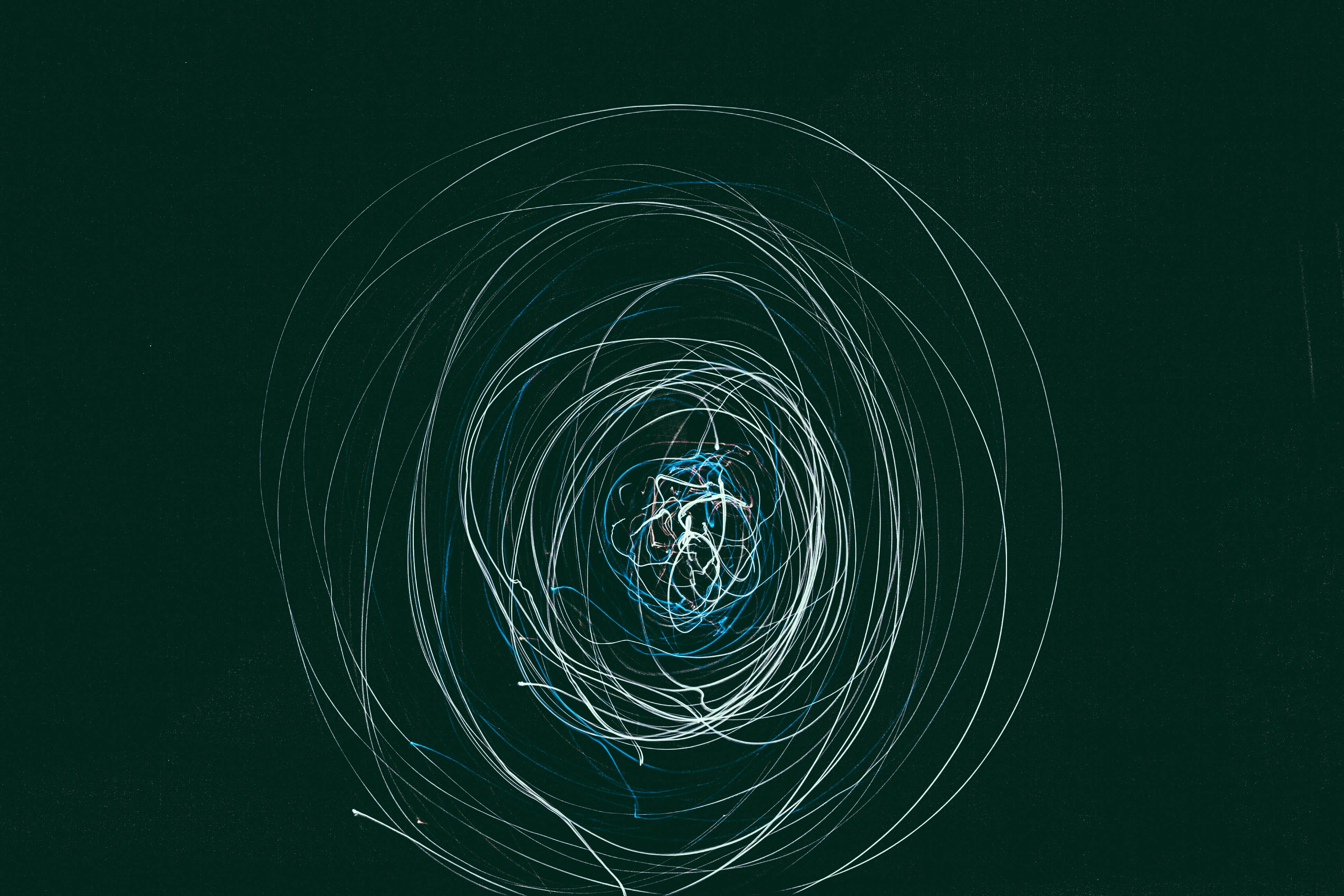(Photo by Gertrūda Valasevičiūtė on Unsplash)

ML is extracting data from knowledge.

Machine learning is a study of algorithms that uses a provides computers the ability to learn from the data and predict outcomes with accuracy, without being explicitly programmed. Machine learning is sub-branched into three categories- supervised learning, unsupervised learning, and reinforcement learning.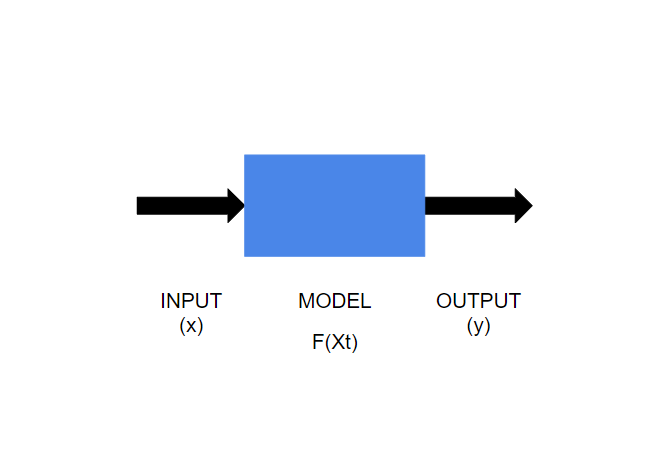(Image by Author) Machine Learning Model

## Supervised learning

As the name "supervised learning" suggests, here learning is based through example. We have a known set of inputs (called features, x) and outputs (called labels, y ). The goal of the algorithm is to train the model on the given data and predict the correct value (y) for an unknown input (x). Supervised learning can be further classified into two categories- classification and regression.

Classification and regression are two basic concepts in supervised learning. However, understanding the difference between the two can be confusing and can lead to the implementation of the wrong algorithm for prediction. If we can understand the difference between the two and identify the algorithm that has to be used, then structuring the model becomes easy.

Classification and regression follow the same basic concept of supervised learning i.e. to train the model on a known dataset to make predict the outcome.

Here the major difference is that in the classification problem the output variable will be assigned to a category or class (i.e. it is discrete), while in regression the variable output is a continuous numerical value.

## Classification

In classification, the model is trained in such a way that the output data is separated into different labels (or categories) according to the given input data.

The algorithm maps the input data (x) to discrete labels (y).

### Binary classification

If there are only two categories in which the given data has to be classified then it is called binary classification. For example- checking a bank transaction whether it is a fraudulent or a genuine transaction. Here, there are only two categories (i.e. fraudulent or genuine) where the output can be labeled.

### Multiclass classification

In this kind of problem, the input is categorized into one class out of three or more classes.

Iris dataset is a perfect example of multiclass classification. Iris data set contains data of fifty samples of three species of flower (setosa, versicolor, and virginica) which are classified based on four parameters (sepal length, sepal width, petal length, and petal width).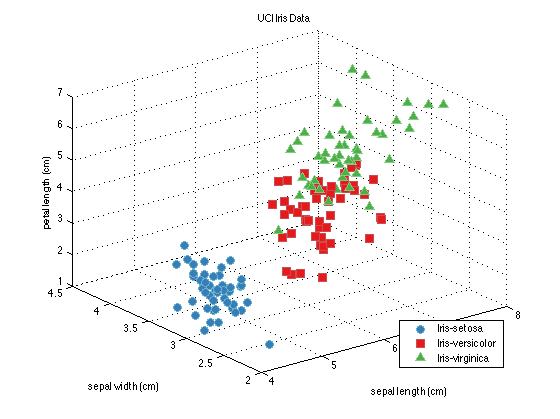(Image by Author) Graphical representation of a linear discriminant model of Iris dataset

### Two Kind of Classifiers

Soft Classifier

A soft classifier predicts the labels for inputs based on the probabilities. For a given input probability for each class (label) is calculated and the input is classified into the class with the highest probability. Higher probability also shows higher accuracy and precision of the model.

The sigmoid function can be used in this model since we have to predict the probabilities. This is because the sigmoid function exists between (0,1) and probability also exists between the same range.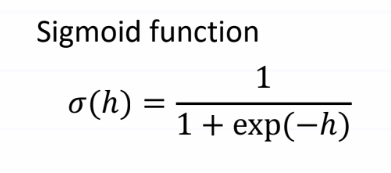(Image by Author) Sigmoid Function

Hard Classifier

Hard classifiers do not calculate the probabilities for different categories and give the classification decision based on the decision boundary.

### Linear and Non- Linear Classifiers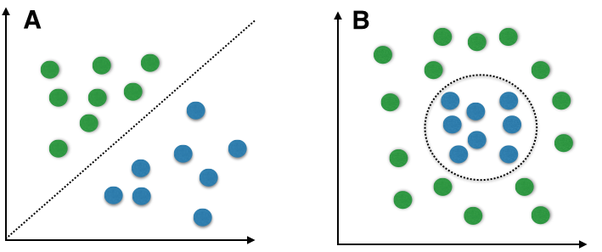(Image by Sebastian Raschka on WikimediaCommons) Graph A represents a linear classifier model. Graph B represents a non-linear classifier model.

Linear Classification Model

When the given data of two classes represented on a graph can be separated by drawing a straight line than the two classes are called linearly separable (in graph A above, green dots and blue dots, these two classes are completely separated by a single straight line).

There can be infinite lines that can differentiate between two classes.

To find the exact position of the line, the type of classifier used is called a linear classifier. Few examples of linear classifiers are- Logistic Regression, Perceptron, Naive Bayes, etcetera.

Non-Linear Classification Model

Here as we can see in graph B (above), two classes cannot be separated by drawing a straight line and therefore requires an alternative way to solve this kind of problem. Here model generates nonlinear boundaries and how that boundary will look like is defined by non-linear classifiers. Few examples of non-linear classifiers are- Decision Trees, K-Nearest Neighbour, Random Forest, etcetera.

## Regression

Unlike classification, here the regression model is trained in such a way that it predicts continuous numerical value as an output based on input variables.

The algorithm maps the input data (x) to continuous or numerical data(y).

There are several kinds of regression algorithms in machine learning like- linear regression, polynomial regression, quantile regression, lasso regression, etc. Linear regression is the simplest method of regression.

### Linear Regression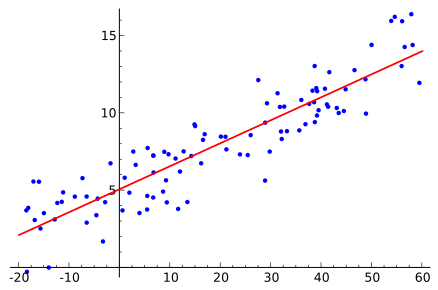(Image by Sewaqu on WikimediaCommons) Graphical Representation of Linear Regression Problem

This approach is generally used for predictive analysis. In this case, a linear relationship is set up between the x-axis feature and the y-axis feature. But as you can see in the graph the line does not pass through every point, but it represents a relationship between the two.

Simple linear regression relation can be represented in the form of an equation as:

y = wx + b

Here, y is numerical output, w is the weight (slope), x is the input variable and b is the bias (or y-intercept).

Regression models can be used in the prediction of temperature, trend forecast, analyze the effect of change of one variable on other variables.

## Conclusion

Supervised learning is the easiest and simplest sub-branch of machine learning. Identification of the correct algorithm to structure the model is very necessary and I hope you are able to understand the difference between regression and classification after reading this article. Try implementing these concepts for better understanding.

If you have any questions or comments, please post them in the comment section.

`Sources:https://developers.google.com/machine-learning/crash-course/ml-introhttps://www.educative.io/edpresso/what-is-the-difference-between-regression-and-classificationhttps://www.statisticssolutions.com/what-is-linear-regression/https://www.geeksforgeeks.org/ml-classification-vs-regression/`

1.1.2.3.4.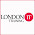Great post about python. Thanks for content writter.

python course london

5.Thanks for Sharing This Article.It is very so much valuable content. I hope these Commenting lists will help to my website
angular js online training
best angular js online training
top angular js online training

6.Thanks for the information. This is very nice blog. Keep posting these kind of posts. All the best.

Machine learning is enabling industrial shifts for increased efficiency. In 2021, the following industries are predicted to adopt machine learning.

In 2021, Machine Learning Is Set To Transform These 5 Industries/Machine Learning, Analytics Insight, Machine Learning in Business, Machine Learning Trends

7.